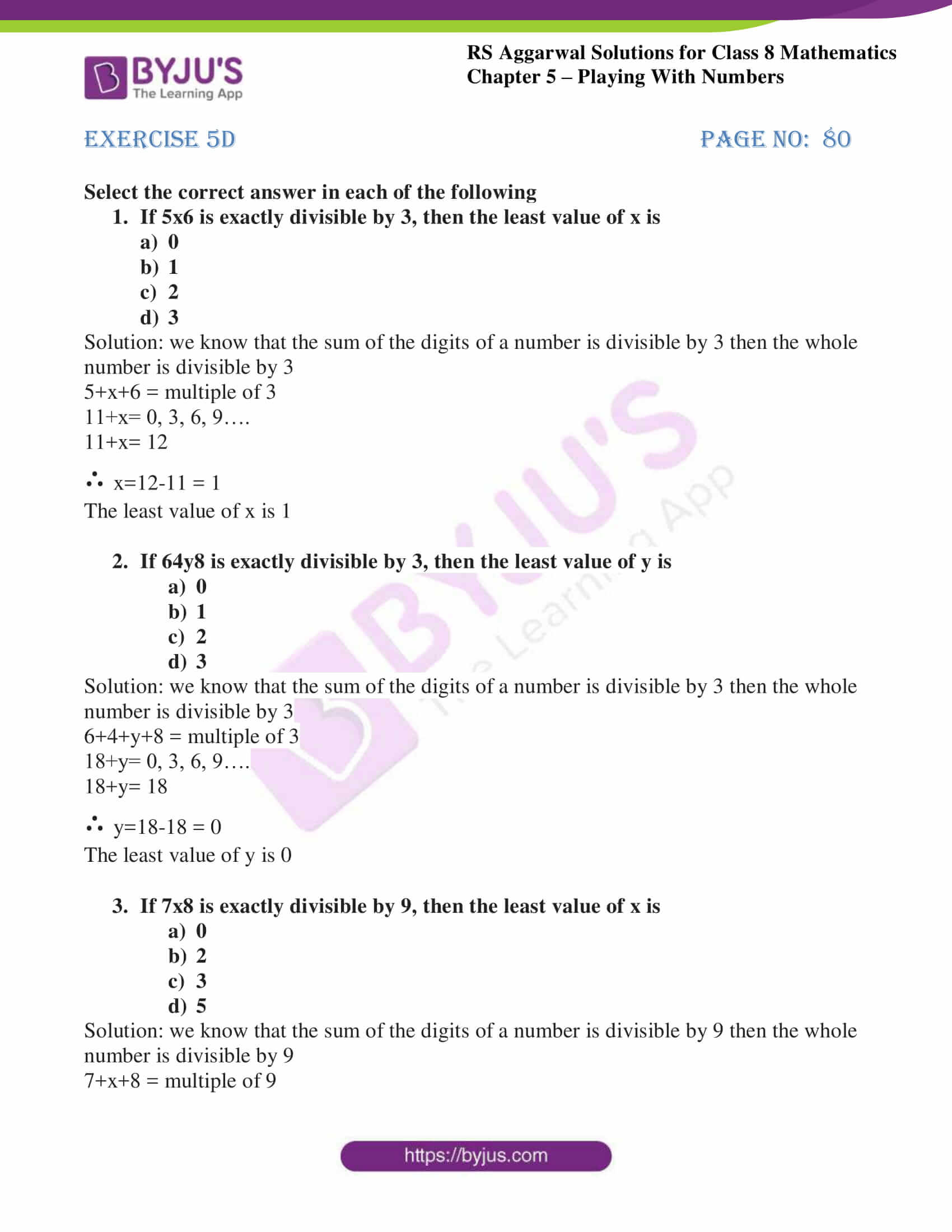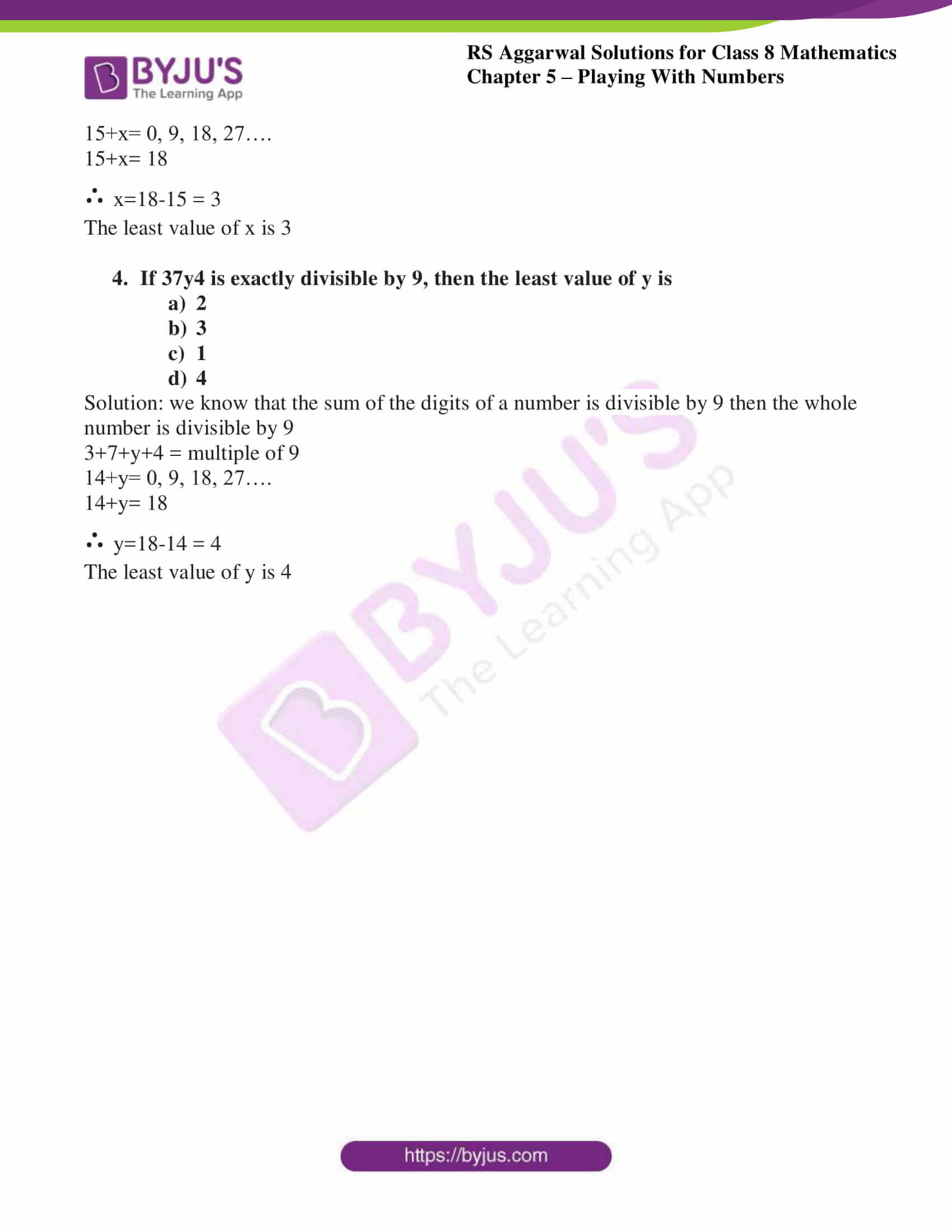# RS Aggarwal Solutions for Class 8 Maths Chapter 5 - Playing with Numbers Exercise 5D

The RS Aggarwal Solutions PDF can be downloaded for Class 8 Maths Chapter 5- Exercise 5D, Playing with Numbers from the links provided below. From the exam point of view, our experts have solved the RS Aggarwal Solutions to ensure that the students are meticulous with their fundamentals by practicing the solutions. In Exercise 5D of RS Aggarwal Class 8 Maths, we shall brief all the concepts covered in this Chapter i.e., the Generalised form, various Tests of Divisibility and replacing alphabets by suitable numerals.

## Download PDF of RS Aggarwal Solutions for Class 8 Maths Chapter 5- Playing with Numbers Exercise 5D### Access Answers to RS Aggarwal Solutions for Class 8 Maths Chapter 5- Playing with Numbers Exercise 5D

1. Select the correct answer in each of the following

1. If 5×6 is exactly divisible by 3, then the least value of x is

a) 0
b) 1
c) 2
d) 3

Solution: we know that the sum of the digits of a number is divisible by 3 then the whole number is divisible by 3

5+x+6 = multiple of 3

11+x= 0, 3, 6, 9….

11+x= 12

∴ x=12-11 = 1

The least value of x is 1

2. If 64y8 is exactly divisible by 3, then the least value of y is

a) 0
b) 1
c) 2
d) 3

Solution: we know that the sum of the digits of a number is divisible by 3 then the whole number is divisible by 3

6+4+y+8 = multiple of 3

18+y= 0, 3, 6, 9….

18+y= 18

∴ y=18-18 = 0

The least value of y is 0

3. If 7×8 is exactly divisible by 9, then the least value of x is

a) 0
b) 2
c) 3
d) 5

Solution: we know that the sum of the digits of a number is divisible by 9 then the whole number is divisible by 9

7+x+8 = multiple of 9

15+x= 0, 9, 18, 27….

15+x= 18

∴ x=18-15 = 3

The least value of x is 3

4. If 37y4 is exactly divisible by 9, then the least value of y is

a) 2
b) 3
c) 1
d) 4

Solution: We know that the sum of the digits of a number is divisible by 9 then the whole number is divisible by 9

3+7+y+4 = multiple of 9

14+y= 0, 9, 18, 27….

14+y= 18

∴ y=18-14 = 4

The least value of y is 4

### Access other Exercises of RS Aggarwal Solutions for Class 8 Maths Chapter 5- Playing with Numbers

Exercise 5A Solutions 6 Questions

Exercise 5B Solutions 15 Questions

Exercise 5C Solutions 14 Questions

## RS Aggarwal Solutions for Class 8 Maths Chapter 5- Playing with Numbers Exercise 5D

Exercise 5D of RS Aggarwal Class 8, Playing with Numbers contains the concepts related to Numbers. This exercise mainly deals with the objective questions related to the entire Chapter 5.

The RS Aggarwal Solutions can help the students practice diligently while learning the fundamentals as it provides all the answers to the questions from the RS Aggarwal textbook. Students are suggested to practice the problems on a regular basis which will help them excel in their exams and increase their overall percentage. Practicing several times helps in achieving high marks and also helps in maximising time management skills.